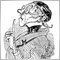# Relation with previous value of Buffer103

Hi all,
I'm learning to program mql4.
I want to ask a simple question because I'm trying to learn the behavior of buffers in one indicator.

If for example, I want to add / subtract the value of the buffer prior Bar an amount according to a certain condition, how I relate to the previous buffer?

```#property strict
#property indicator_separate_window
#property indicator_buffers 1
double a[];

int init()

{

SetIndexBuffer(0,a);
SetIndexStyle(0,DRAW_LINE,STYLE_SOLID,2,clrRed);

return(0);

}

int start()

{

double b;

int counted_bars=IndicatorCounted();

if (counted_bars>0)
counted_bars--;

int limit = Bars-counted_bars;

for (int i=0;i<limit;i++)

{

double c1=iClose(NULL,0,i);
double c2=iOpen(NULL,0,i);

if(c1>c2){

b=a[i+1];
a[i]=b+c1;
}
else
{

b=a[i+1];
a[i]=b-c1;
}

}

return(0);

}  ```

Thank you very much in advance,

I hope, i explained well the situation!!.

Any suggest is good for to leanModerator
10120

You are looking for:

a[i] = a[i+1] + (condition ? value1 : value2);

?103

gooly:
You are looking for:

a[i] = a[i+1] + (condition ? value1 : value2);

?

Yes,

if close is higher than open, then

i summ to current close the previous "a".

This is the logic, but something i am having wrongModerator
10120

castann86:

Yes,

if close is higher than open, then

i summ to current close the previous "a".

This is the logic, but something i am having wrong

Make sure that you count down the bar-index from Bars-n .. 0! Otherwise you'll  create a useless repainting indicator103

The indicator is only for learn the relation between buffers and your behavior.

The indicator have to draw line in separate window, but i think i am not refering well to previous value of buffer

Thank you very much!103

any suggest ?

thank you very much22339

```if(c1>c2){      // An up candle
b=a[i+1];    // previous value
a[i]=b+c1;   // what is the meaning of Close[x+1] + Close[x]?
// E.g. Close=1.5000 Close= 1.5010 Close=1.5020 a=4.503 meaningless```103

Let me make it easy explanation. It is only for learn the behavior in mql4.

For example:

a[i+1] = 10

and each step i want to add 2 units :

a[i]=a[i+1]+2;

So, in the above code of first post:

If ( current close is higher than current open) then

b is equal to previous value of buffer "a"

Current value of buffer is equal to "b" plus current close.

I writte in this way because maybe i not explain correctly or there is any mystake in the logic.

This is for learn relationship between buffers, not make sense this code for any indicator.

Thank you very much. Some suggest can be very usefull.22339

1. You aren't adding 2 to a buffer you are adding the close to the buffer then another close to the buffer...
2. When prices are 1.5xxx adding 2 to it is meaningless.103

Sorry, forget the code at this time,

if current value of buffer is equal to previous value of buffer plus one number(any number).

Can you put a example of this statement?

I am not searching to code a indicator. I search how can i relationship a previous value of one buffer with current value of same buffer.

Sorry for my wrong explanation.